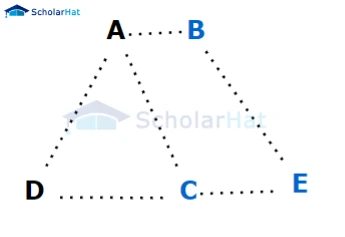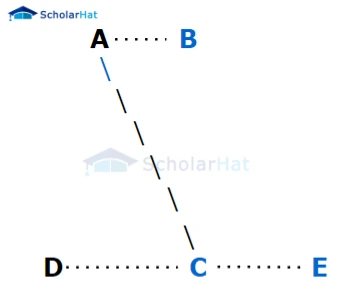# Spanning Tree in Data Structures

Amit Kumar Ghosh  10 min read
19 Jun 2023
304 Views

## Introduction

Have you ever developed a data structure and asked yourself, what is the best way to make sure all the nodes in your network are connected? Spanning tree algorithms can offer an answer. Spanning tree algorithms help determine which connections are necessary for each node in your network so that it becomes fully connected with minimum cost. In this article, we’ll explore exactly how these algorithms work as well as their benefits to a data structure. We’ll also provide advice on when and where they should be used within a project. So if you're looking for a way to streamline your data structures by finding optimal routes between nodes, keep reading!

## What is Spanning Tree in Data Structure?

In the wide field of data structures, spanning tree is a crucial aspect for the effective organization of data. It is essentially a tree-like structure that is derived from a connected graph. The spanning tree in data structure is designed to cover all the vertices of the graph with a minimal amount of edges, creating a simplified representation of the original complex network. This structure is commonly used in various applications such as the creation of routing tables, network optimization, and even in mapping algorithms. Understanding the concept of spanning trees is fundamental to ensuring a stable and efficient data management system.

Here's a step-by-step process to visualize a spanning tree:

• Original graph:Step 1: Choose a vertex as the root of the spanning tree. Let's select vertex A as the root.
Step 2: Perform a depth-first search (DFS) or breadth-first search (BFS) starting from the root to traverse the graph and create the spanning tree. During the traversal, only select edges that have not been visited before and do not create a cycle. Starting from A, explore its unvisited neighbors in alphabetical order (B and D).

Step 3: Select the edge A-B, mark it as part of the spanning tree, and proceed to vertex B.Step 4: From B, explore its unvisited neighbor C. Select the edge B-C, mark it as part of the spanning tree, and proceed to vertex C.Step 5: From C, explore its unvisited neighbors D and E. Select the edge C-D, mark it as part of the spanning tree, and proceed to vertex D.Step 6: From D, there are no unvisited neighbors, so backtrack to C.

Step 7: From C, explore its unvisited neighbor E. Select the edge C-E, mark it as part of the spanning tree, and proceed to vertex E.Step 8: From E, there are no unvisited neighbors, so backtrack to C.

Step 9: From C, there are no unvisited neighbors, so backtrack to B.

Step 10: From B, there are no unvisited neighbors, so backtrack to A.

Step 11: Traversal is complete, and the resulting subgraph forms the spanning tree of the original graph. Spanning tree:This spanning tree includes all the vertices (A, B, C, D, and E) and forms a tree-like structure without any cycles. The edges (A-B), (B-C), (C-D), and (C-E) are part of the spanning tree, while the remaining edges are not.

## Application of Spanning Tree

1. Network Design: In network engineering, spanning trees are used to construct efficient and fault-tolerant network topologies. Spanning tree algorithms, such as the Spanning Tree Protocol (STP) and its variants, help to eliminate loops in Ethernet networks and ensure that there is a single path between any two nodes in the network.
2. Broadcast Storm Prevention: In computer networks, broadcast storms can occur when a broadcast or multicast packet circulates endlessly through a network, consuming excessive bandwidth and causing network congestion. Spanning tree algorithms help prevent broadcast storms by identifying and blocking redundant paths in the network, thereby creating a loop-free topology.
3. Routing: Spanning trees can be used as a basis for routing algorithms. By constructing a minimum spanning tree (MST) of a network, it is possible to determine the most efficient path between any two nodes. This information can be utilized in routing protocols to make intelligent decisions on forwarding packets through the network.
4. Network Monitoring: Spanning trees can be employed for network monitoring and analysis. By capturing the network traffic on each link of the spanning tree, it becomes easier to monitor the performance, identify bottlenecks, and analyze network behavior. This information is valuable for troubleshooting, capacity planning, and optimizing network performance.
5. Redundancy and High Availability: Spanning trees allow for the creation of redundant links in a network. By utilizing redundant links, network administrators can ensure high availability and fault tolerance. In the event of a link or switch failure, the spanning tree algorithm dynamically reroutes traffic through alternative paths, minimizing downtime and maintaining connectivity.
6. Sensor Networks: Spanning trees find applications in wireless sensor networks (WSNs), where sensors are deployed to gather data in various environments. By constructing a spanning tree in a WSN, energy consumption can be minimized as only a subset of sensors needs to transmit data to a central node, reducing redundant transmissions and prolonging the network lifetime.
7. Clustering and Hierarchical Structures: Spanning trees can be utilized in data analysis and clustering algorithms. By constructing a minimum spanning tree of a dataset, it is possible to identify hierarchical structures and clustering patterns within the data, providing insights into the relationships between data points.

## Properties of Spanning Tree

1. Connectivity: A spanning tree must connect all the vertices of the original graph. This means that there is a path between any two vertices in the spanning tree.
2. Acyclicity: A spanning tree is an acyclic graph, which means it contains no cycles. There are no closed loops or circuits in a spanning tree.
3. The number of edges: A spanning tree contains exactly (V - 1) edges, where V is the number of vertices in the original graph. Adding any additional edge would introduce a cycle and violate the tree property.
4. Minimal connected subgraph: A spanning tree is the smallest connected subgraph that can be formed from the original graph while including all of its vertices. Removing any edge from the spanning tree would disconnect the graph.
5. Tree structure: As a tree, a spanning tree has no loops or circuits. It is a connected graph with no cycles, and there is a unique path between any two vertices.
6. Preservation of connectivity: A spanning tree preserves the connectivity of the original graph. This means that if there is a path between two vertices in the original graph, there will also be a path between them in the spanning tree.
7. Spanning tree weight: If the original graph is weighted, where each edge has a numerical weight, the weight of a spanning tree is defined as the sum of the weights of all its edges. There may be multiple spanning trees for a given graph, and their weights can vary.

## Mathematical Properties of Spanning Tree

1. The number of edges in a spanning tree is equal to the number of nodes (vertices) minus one.
2. A spanning tree can be constructed from a complete graph by removing a maximum of e - n + 1 edges.
3. The maximum number of spanning trees in a complete graph is equal to n raised to the power of n-2.

## Minimum Spanning Tree Algorithm

A minimum spanning tree algorithm (MST) is a subset of the edges of a connected, weighted graph that connects all the vertices together with the minimum total edge weight. In other words, it is a tree that spans all the vertices of the graph with the minimum possible sum of edge weights. The concept of a minimum spanning tree is often used in network design, where the goal is to connect a set of nodes together at the least cost. It has applications in various fields such as transportation networks, computer networks, and telecommunications.

## Algorithms for Minimum Spanning Tree

The Minimum Spanning Tree (MST) algorithm is a widely used algorithm in graph theory and computer science. It finds the minimum spanning tree of a connected, weighted graph. A minimum spanning tree is a tree that connects all the vertices of the graph with the minimum total edge weight.

There are different algorithms to find the minimum spanning tree, but two of the most popular ones are:

### Kruskal's Algorithm:

• Sort all the edges of the graph in the non-decreasing order of their weights.
• Initialize an empty graph, which will be the minimum spanning tree.
• Iterate over the sorted edges. For each edge, if adding it to the current tree does not create a cycle, add it to the tree.
• Continue this process until there are (V-1) edges in the tree, where V is the number of vertices in the original graph.

### Prim's Algorithm:

• Choose a starting vertex.
• Initialize a priority queue (min-heap) to store the edges with their weights.
• Add all the edges connected to the starting vertex to the priority queue.
• While the priority queue is not empty, remove the edge with the minimum weight from the queue.
• If the removed edge connects to a vertex that has not been visited yet, add it to the minimum spanning tree and mark the vertex as visited.
• Add all the edges connected to the newly visited vertex to the priority queue.
• Continue this process until all vertices have been visited.

Both algorithms guarantee to find the minimum spanning tree of a connected, weighted graph. Kruskal's algorithm has a time complexity of O(E log E), where E is the number of edges, while Prim's algorithm has a time complexity of O(E log V), where V is the number of vertices.

##### Summary

In conclusion, a spanning tree in the data structure is an essential data structure that offers the advantage of locating connections quickly and within a limited search space. It allows for efficient intra-connectivity while maintaining the minimalist tree structure, making it useful for environments where merging data points is a common activity. Its overall flexibility makes it a great fit for large-scale projects and complex networks, helping to reduce the time spent creating links between multiple nodes. So if you have been considering adding a spanning tree in data structure applications to your project, do yourself a favour and give it a try you won’t be disappointed!

Similar Articles